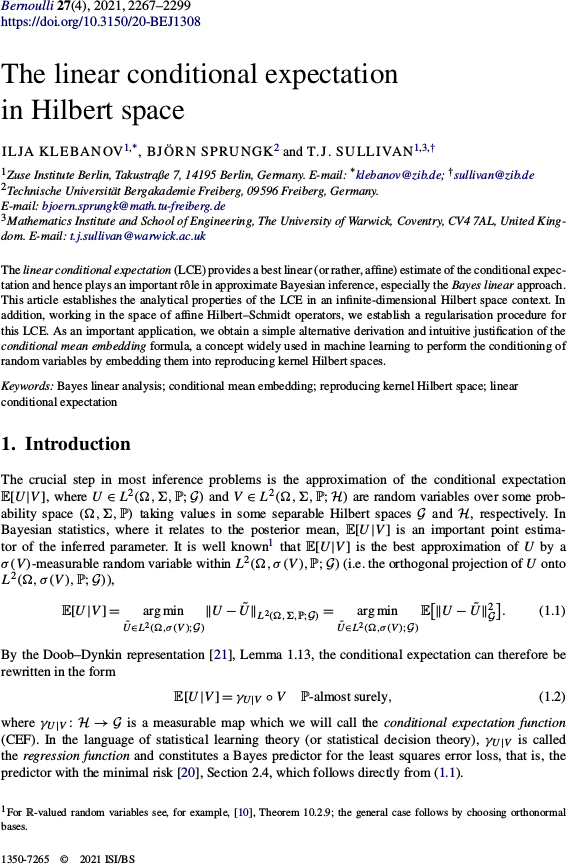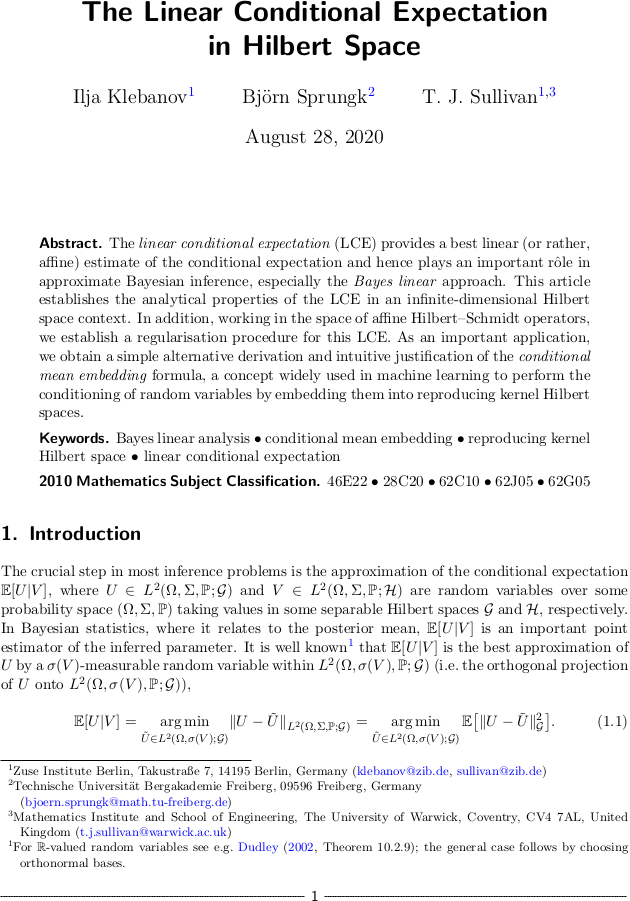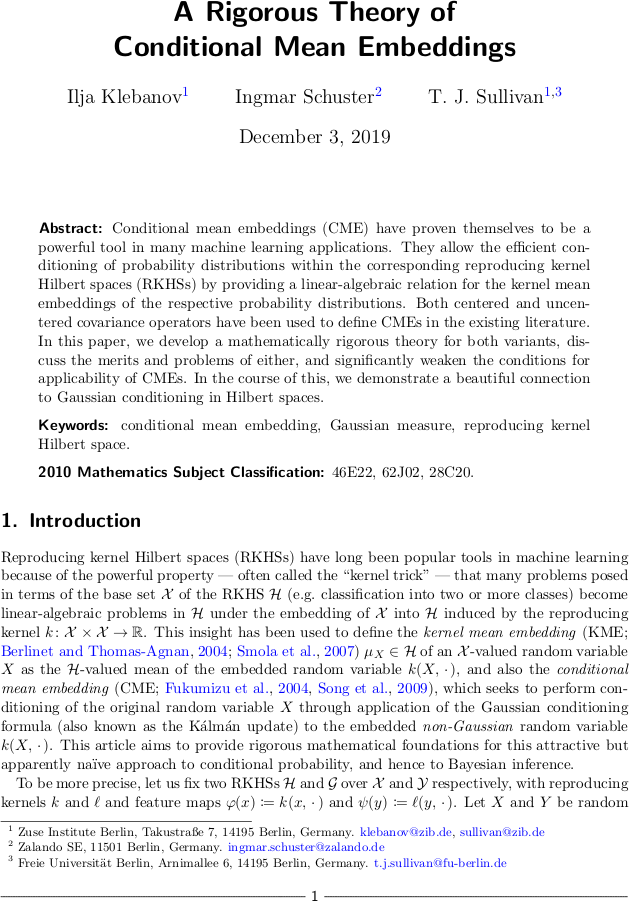# Tim Sullivan

### #mean-embedding

Clear Search### Linear conditional expectation in Hilbert space in Bernoulli

The article “The linear conditional expectation in Hilbert space” by Ilja Klebanov, Björn Sprungk, and myself has just appeared in its final form in the journal Bernoulli. In this paper, we study the best approximation $$\mathbb{E}^{\mathrm{A}}[U|V]$$ of the conditional expectation $$\mathbb{E}[U|V]$$ of an $$\mathcal{G}$$-valued random variable $$U$$ conditional upon a $$\mathcal{H}$$-valued random variable $$V$$, where “best” means $$L^{2}$$-optimality within the class $$\mathrm{A}(\mathcal{H}; \mathcal{G})$$ of affine functions of the conditioning variable $$V$$. This approximation is a powerful one and lies at the heart of the Bayes linear approach to statistical inference, but its analytical properties, especially for $$U$$ and $$V$$ taking values in infinite-dimensional spaces $$\mathcal{G}$$ and $$\mathcal{H}$$, are only partially understood — which this article aims to rectify.

I. Klebanov, B. Sprungk, and T. J. Sullivan. “The linear conditional expectation in Hilbert space.” Bernoulli 27(4):2267–2299, 2021. doi:10.3150/20-BEJ1308

Abstract. The linear conditional expectation (LCE) provides a best linear (or rather, affine) estimate of the conditional expectation and hence plays an important rôle in approximate Bayesian inference, especially the Bayes linear approach. This article establishes the analytical properties of the LCE in an infinite-dimensional Hilbert space context. In addition, working in the space of affine Hilbert–Schmidt operators, we establish a regularisation procedure for this LCE. As an important application, we obtain a simple alternative derivation and intuitive justification of the conditional mean embedding formula, a concept widely used in machine learning to perform the conditioning of random variables by embedding them into reproducing kernel Hilbert spaces.

Published on Wednesday 25 August 2021 at 08:00 UTC #publication #tru2 #bayesian #rkhs #mean-embedding #klebanov #sprungk### Linear conditional expectation in Hilbert space

Ilja Klebanov, Björn Sprungk, and I have just uploaded a preprint of our recent work “The linear conditional expectation in Hilbert space” to the arXiv. In this paper, we study the best approximation $$\mathbb{E}^{\mathrm{A}}[U|V]$$ of the conditional expectation $$\mathbb{E}[U|V]$$ of an $$\mathcal{G}$$-valued random variable $$U$$ conditional upon a $$\mathcal{H}$$-valued random variable $$V$$, where “best” means $$L^{2}$$-optimality within the class $$\mathrm{A}(\mathcal{H}; \mathcal{G})$$ of affine functions of the conditioning variable $$V$$. This approximation is a powerful one and lies at the heart of the Bayes linear approach to statistical inference, but its analytical properties, especially for $$U$$ and $$V$$ taking values in infinite-dimensional spaces $$\mathcal{G}$$ and $$\mathcal{H}$$, are only partially understood — which this article aims to rectify.

Abstract. The linear conditional expectation (LCE) provides a best linear (or rather, affine) estimate of the conditional expectation and hence plays an important rôle in approximate Bayesian inference, especially the Bayes linear approach. This article establishes the analytical properties of the LCE in an infinite-dimensional Hilbert space context. In addition, working in the space of affine Hilbert–Schmidt operators, we establish a regularisation procedure for this LCE. As an important application, we obtain a simple alternative derivation and intuitive justification of the conditional mean embedding formula, a concept widely used in machine learning to perform the conditioning of random variables by embedding them into reproducing kernel Hilbert spaces.

Published on Friday 28 August 2020 at 09:00 UTC #preprint #tru2 #bayesian #rkhs #mean-embedding #klebanov #sprungk### A rigorous theory of conditional mean embeddings in SIMODS

The article “A rigorous theory of conditional mean embeddings” by Ilja Klebanov, Ingmar Schuster, and myself has just appeared online in the SIAM Journal on Mathematics of Data Science. In this work we take a close mathematical look at the method of conditional mean embedding. In this approach to non-parametric inference, a random variable $$Y \sim \mathbb{P}_{Y}$$ in a set $$\mathcal{Y}$$ is represented by its kernel mean embedding, the reproducing kernel Hilbert space element

$$\displaystyle \mu_{Y} = \int_{\mathcal{Y}} \psi(y) \, \mathrm{d} \mathbb{P}_{Y} (y) \in \mathcal{G},$$

and conditioning with respect to an observation $$x$$ of a related random variable $$X \sim \mathbb{P}_{X}$$ in a set $$\mathcal{X}$$ with RKHS $$\mathcal{H}$$ is performed using the Woodbury formula

$$\displaystyle \mu_{Y|X = x} = \mu_Y + (C_{XX}^{\dagger} C_{XY})^\ast \, (\varphi(x) - \mu_X) .$$

Here $$\psi \colon \mathcal{Y} \to \mathcal{G}$$ and $$\varphi \colon \mathcal{X} \to \mathcal{H}$$ are the canonical feature maps and the $$C$$'s denote the appropriate centred (cross-)covariance operators of the embedded random variables $$\psi(Y)$$ in $$\mathcal{G}$$ and $$\varphi(X)$$ in $$\mathcal{H}$$.

Our article aims to provide rigorous mathematical foundations for this attractive but apparently naïve approach to conditional probability, and hence to Bayesian inference.

I. Klebanov, I. Schuster, and T. J. Sullivan. “A rigorous theory of conditional mean embeddings.” SIAM Journal on Mathematics of Data Science 2(3):583–606, 2020. doi:10.1137/19M1305069

Abstract. Conditional mean embeddings (CMEs) have proven themselves to be a powerful tool in many machine learning applications. They allow the efficient conditioning of probability distributions within the corresponding reproducing kernel Hilbert spaces by providing a linear-algebraic relation for the kernel mean embeddings of the respective joint and conditional probability distributions. Both centered and uncentered covariance operators have been used to define CMEs in the existing literature. In this paper, we develop a mathematically rigorous theory for both variants, discuss the merits and problems of each, and significantly weaken the conditions for applicability of CMEs. In the course of this, we demonstrate a beautiful connection to Gaussian conditioning in Hilbert spaces.

Published on Wednesday 15 July 2020 at 08:00 UTC #publication #simods #mathplus #tru2 #rkhs #mean-embedding #klebanov #schuster### A rigorous theory of conditional mean embeddings

Ilja Klebanov, Ingmar Schuster, and I have just uploaded a preprint of our recent work “A rigorous theory of conditional mean embeddings” to the arXiv. In this work we take a close mathematical look at the method of conditional mean embedding. In this approach to non-parametric inference, a random variable $$Y \sim \mathbb{P}_{Y}$$ in a set $$\mathcal{Y}$$ is represented by its kernel mean embedding, the reproducing kernel Hilbert space element

$$\displaystyle \mu_{Y} = \int_{\mathcal{Y}} \psi(y) \, \mathrm{d} \mathbb{P}_{Y} (y) \in \mathcal{G},$$

and conditioning with respect to an observation $$x$$ of a related random variable $$X \sim \mathbb{P}_{X}$$ in a set $$\mathcal{X}$$ with RKHS $$\mathcal{H}$$ is performed using the Woodbury formula

$$\displaystyle \mu_{Y|X = x} = \mu_Y + (C_{XX}^{\dagger} C_{XY})^\ast \, (\varphi(x) - \mu_X) .$$

Here $$\psi \colon \mathcal{Y} \to \mathcal{G}$$ and $$\varphi \colon \mathcal{X} \to \mathcal{H}$$ are the canonical feature maps and the $$C$$'s denote the appropriate centred (cross-)covariance operators of the embedded random variables $$\psi(Y)$$ in $$\mathcal{G}$$ and $$\varphi(X)$$ in $$\mathcal{H}$$.

Our article aims to provide rigorous mathematical foundations for this attractive but apparently naïve approach to conditional probability, and hence to Bayesian inference.

Abstract. Conditional mean embeddings (CME) have proven themselves to be a powerful tool in many machine learning applications. They allow the efficient conditioning of probability distributions within the corresponding reproducing kernel Hilbert spaces (RKHSs) by providing a linear-algebraic relation for the kernel mean embeddings of the respective probability distributions. Both centered and uncentered covariance operators have been used to define CMEs in the existing literature. In this paper, we develop a mathematically rigorous theory for both variants, discuss the merits and problems of either, and significantly weaken the conditions for applicability of CMEs. In the course of this, we demonstrate a beautiful connection to Gaussian conditioning in Hilbert spaces.

Published on Tuesday 3 December 2019 at 07:00 UTC #preprint #mathplus #tru2 #rkhs #mean-embedding #klebanov #schuster